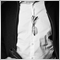# XOR in mql4 - page 21178

JeeyCi #:

answer #3 seems to be the best - with x & y as conditions & b as overall result (as for XOR)... with such a tip (^) no any violation of XOR logics was noticed by me in real coding in MQL

The issue lies within the type of operation to be performed.

logical operators "do not" support XOR as a separate type, it is represented with unequal, as shown.

Let me give you an example of arithmetic/bitwise and logical operation:

```const int A = 1;
const int B = 3;

const int arithmetic_result = A^B;                              // = 2;
const bool logical_result = ((A != B)                           // = true;
cosnt bool type_safe_result = (((bool)A) != ((bool)B));         // = false

```

As you can see, the interpretation depends on the type.

So when the code is using logical operators "&&", the result is different to the bitwise/arithmetic approach.

This is an important distinguishment.1178

Dominik Egert #:
XOR = A != B

My mistake in this reply was not to give the types, as I interpreted them to be bool.

In fact, if not specified, mql compiler defaults to type "int", since there is no type giving operator in place. This is different for the statement

`const bool type_specific_result = (!A&&B)||(A&&!B);`

Here the type is given by the logical operator, interpreting the values A and B as logical "true" and "false" values. Since the "&&" operator is specific to the type "bool".